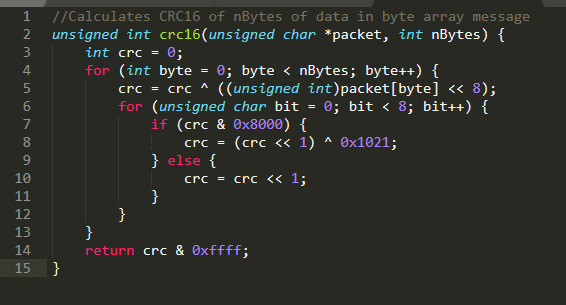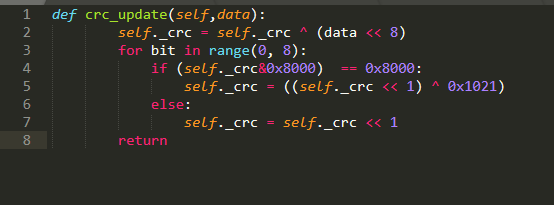# Introduction

When data is transmitted between digital system there is a need to know that the information shared hasn’t been corrupted in transit. There are several ways to do this, however the RoboClaw uses a method known as a Cyclic Redundancy Check.

# What, Why and How of the CRC

The CRC is a set of mathematical operations that takes data and produces a numerical value that can be used to verify the integrity of the message after it has been sent or received. When the data is sent the CRC value is added to the end of the message. The receiver can then perform the same set of operations and calculate the CRC. It the two CRC values are the same the data is valid, if not something about the data is incorrect and should not be used. The CRC will detect changes as small as a single bit, so any error in the data will be caught by comparing CRC values.

When data is being sent from one system to another there are a quite a few ways in which the data being sent can be changed inadvertently. Packets on a network may be lost, bits in a message may be flipped or lost, or fluctuations in power may corrupt data while it is being transferred.

Here is an example of calling the CRC C code posted below:

int value = crc16(“Hello World”, 11);

printf(“%x”, value);

The result as printed is: 992a

The CRC is calculated by moving one byte of the message in at a time. Each byte is XOR’d with the current CRC value, with the intial value of the CRC being 0. Before being XOR’d the byte is shifted left by 8 bits. After the calculation on the whole byte is done the indivdual bits of the byte are handled. If the highest bit of the CRC is a non-zero value the CRC value is left shifted by one bit and XOR’d with the unique key for the CRC-16 algorithm in use, in the CRC algorithm we use the value of the key is 0x1021. If the leading bit of the CRC is a zero the whole CRC value is shifted left one bit. This process continues until all the bytes of the message have been processed. Once this is complete the final CRC value has been calculated and can be used.

Once the CRC has been calculated it can be sent along with your data to the RoboClaw. The RoboClaw will then perform the same CRC calculation to confirm it received your data intact. You will also receive a calculated CRC from the RoboClaw when it sends you data. You can then compute the CRC of the received data to verify it’s integrity.

# C

Our code sample below demonstrate how we calculate the CRC. First a byte is moved in to memory and the low part of the byte is shifted to the high part of the byte. It is then XORd with the current CRC value. The initial value of the CRC is 0. After this is done each individual bit is dealt with in order. A check is performed to see if the highest bit of the CRC is a non-zero value, if it is the bit is left shifted and XOR’d with the key in use for the particular flavor of CRC. If the highest bit is a zero a left shift of 1 is performed. This process repeats until the whole CRC has been calculated.Figure 1: CRC calculation in C.

# Python

This Python example work in a similar fashion to the C code above. The main difference is that it works by taking in a byte at time rather than the whole message at a time. Once again the byte is shifted up by 8 bits and XOR’d with the current CRC value. Then each bit is handled conditionally based upon the leading value of the current CRC value and if appropriate XOR’d with the key of 0x1021.Figure 2: CRC calcualtion in Python.